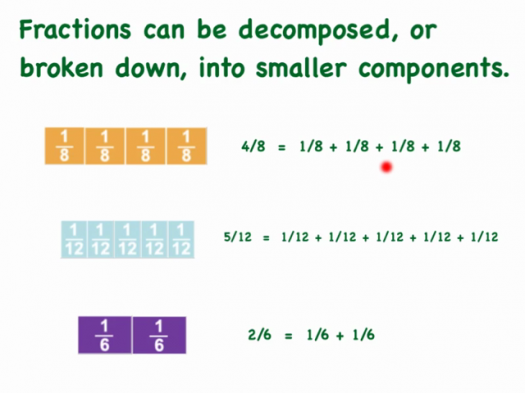# What Do You Know About Decomposing Fractions?

10 Questions | Total Attempts: 113SettingsTo decompose a fraction simply means to take it apart. The most basic way todecompose a fraction is to break into unit fractions, which is when the numerator (top number) is 1. We can see that 5/8 is the same as the unit fraction 1/8 five times.

• 1.
Decomposing Fraction is a topic in which of the following?
• A.

Maths

• B.

Physics

• C.

Chemistry

• D.

Biology

• 2.
To decompose a fraction simply means to ....?
• A.

To bring it together

• B.

Take it apart

• C.

Make it equal

• D.

None

• 3.
The most basic way to decompose a fraction is to break into ....?
• A.

Unit fraction

• B.

Decimal

• C.

Numerator

• D.

Denominator

• 4.
The most basic way to decompose a fraction is to break into unit fraction, which is when the numerator (top number) is ...?
• A.

3

• B.

1

• C.

2

• D.

4

• 5.
We can see that 5/8 is the same as the unit fraction .....?
• A.

2/8

• B.

3/8

• C.

1/8

• D.

8/8

• 6.
We can see that 5/8 is the same as the unit fraction 1/8 how many times?
• A.

Four times

• B.

Two times

• C.

Eight times

• D.

Five times

• 7.
Mathematics is taught in ....?
• A.

Restaurant

• B.

Home

• C.

School

• D.

Museum

• 8.
It is a topic under which subject or course?
• A.

Music

• B.

Literature

• C.

Mathematics

• D.

Biology

• 9.
Decomposing Fractions has nothing to do with the following
• A.

Making equal

• B.

Bringing together

• C.

None

• D.

All of the above

• 10.
Which of the following is a scientist?
• A.

Albert Einstein

• B.

Ellen Poe

• C.

Aliko Dangote

• D.

Barack Obama

Related TopicsBack to top